# Clustering algorithms

## Notation

•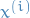is the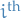observation
•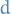is the dimension of the data, i.e.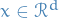## K-means clustering

### Summary

• Guarantees local minima converges
• No guarantee for global minima

### Notation

•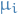is the mean of thecluster

### Idea

K-means clustering is performing using two steps:

1. Randomly initialize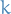cluster centroids with means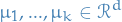1
2. Repeat until convergence:

1. Set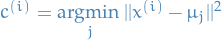, i.e. assign thedata to the cluster for which it is closest to the mean.
2. Update mean of cluster as follows:μj = { {} 1 \{c(i) = j \} x(i)} {{} 1 \{c(i) = j\} }

Convergence here means until the assignments to the different clusters do not change.

## Gaussian mixture models

### Notation

•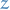is the latent / unobserverd / hidden random variable which we want to estimate
•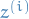is the assigned cluster for theobservation•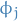represents the probability of the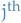"cluster" assignment

### Idea

We have a latent random variableand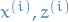have a joint distribution: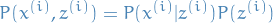2

The model is as follows:

•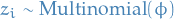where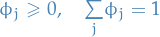•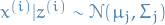Note that this model is identical to Gaussian Discriminant Analysis, except the fact that we have replaced our labeled outputs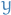by latent variable.

### Optimization

#### Special case of EM

See this for more on Expectation Maximization (EM).

Repeat until convergence:

1. (E-step) Guess values of's and compute: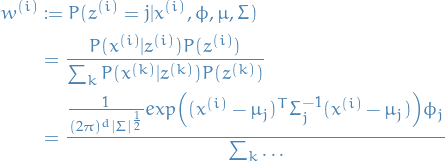3

Where the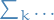means that we sum over the same expression as in the numerator for every, and the numerator is simply the Gaussian PDF multiplied by the probability of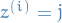.

2. (M-step) Compute for each cluster:
•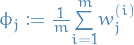, i.e. take average of weights computed above
•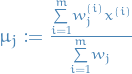, i.e. weighted average for the ith observation
•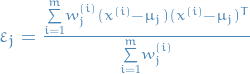, i.e. the variance using the previously computed weights

#### Parallels to Gaussian Discriminant Analysis

In GDA we have: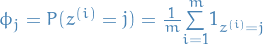But in our case (GMM) we do not know which cluster the observation belongs to, so instead we use the "soft-weights"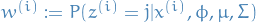and so get.

## Mean-shift

• Non-parametric feature-space analysis technique for locating a maxima of a density function, a so-called mode-seeking algorithm

Mean shift is an iterative method for locating the maxima - the modes - of density function given discrete data sampled from that function.

1. Choose a kernel function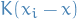, which determines the weights of the nearby points for re-estimation of the mean.
2. The weighted mean of the density in the window determined by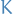is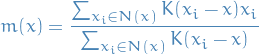where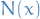is the neighborhood of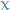, a set of points for which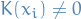.

3. Set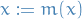4. Repeate 2-3 until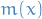converges

The procedure gets it's name from the difference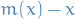, which is called the mean shift.

A rigid proof for convergence of the algorithm using a general kernel in a high dimensional space is still not known.

Convergence has been shown for one-dimension with differentiable, convex, and strictly increasing profile function.

Convergence in higher dimensions with a finite number of the stationary points (or isolated) has been proven, however, sufficient conditions for a general kernel to have a finite (or isolated) stationary points have not been provided.